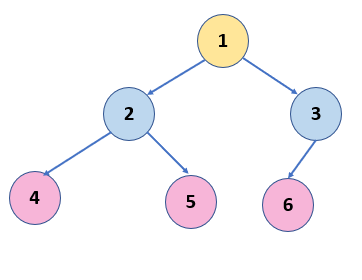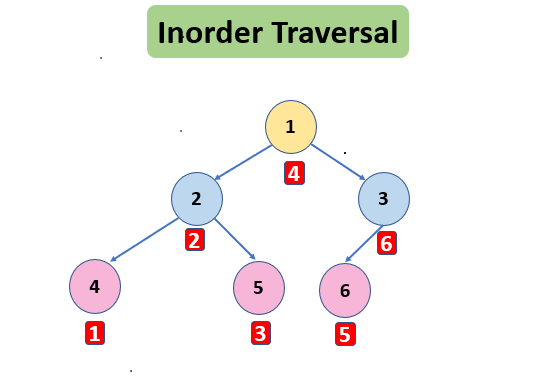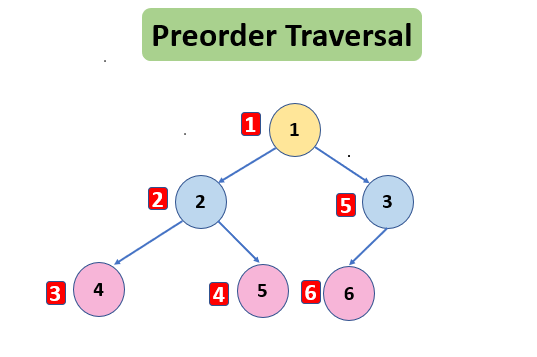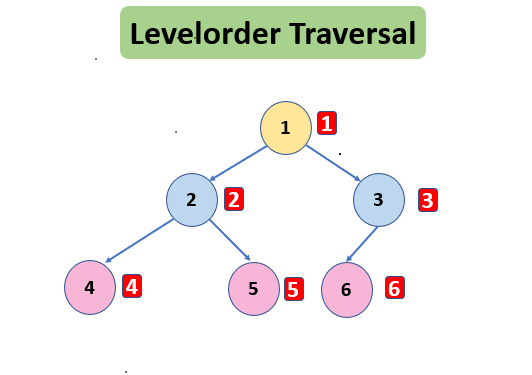# Binary Tree Traversal : Inorder Preorder Postorder

The tree is a non-linear data structure, unlike Linked List and Arrays. It is a hierarchical data structure that can be traversed in the following ways:-

1. Inorder Traversal
2. Preorder Traversal
3. Postorder Traversal
4. Level Order Traversal.

For the given Tree,## Inorder Traversal

Inorder Traversal is one of the tree traversals in which the left subtree is visited first then visit the root and then the right subtree is visited.Inorder Traversal –  4 2 5 1 6 3

Algorithm of Inorder Traversal

1. Traverse the left subtree
2. Print the root
3. Traverse the right subtree

Implementation of Inorder Traversal

## C++

``````struct node {
int data;
struct node * left, * right;
};

void inOrderTrav(node * curr, vector < int > & inOrder) {
if (curr == NULL)
return;

inOrderTrav(curr -> left, inOrder);
inOrder.push_back(curr -> data);
inOrderTrav(curr -> right, inOrder);
}``````

## Java

``````class Node {
int data;
Node left, right;
Node(int data) {
this.data = data;
left = null;
right = null;
}
}

class TUF {

static void inOrderTrav(Node curr, ArrayList < Integer > inOrder) {
if (curr == null)
return;

inOrderTrav(curr.left, inOrder);
inOrderTrav(curr.right, inOrder);
}
}``````

Read Inorder Traversal in details.

Applications of Inorder Traversal

• If inorder traversal of Binary Search Tree (BST) is done , we get increasing order.
• We can get reversed order / Decreasing order by doing inorder traversal in reverse order ( where right subtree is called first and then left subtree is called)

## Preorder Traversal

Preorder Traversal is one of the tree traversals in which root is visited then the left subtree is visited and then the right subtree is visited.Preorder Traversal – 1 2 4 5 3 6

Algorithm of Preorder Traversal

1. Print the root
2. Traverse the left subtree
3. Traverse the right subtree

Implementation of Preorder Traversal

## C++

``````struct node {
int data;
struct node * left, * right;
};

void preOrderTrav(node * curr, vector < int > & preOrder) {
if (curr == NULL)
return;

preOrder.push_back(curr -> data);
preOrderTrav(curr -> left, preOrder);
preOrderTrav(curr -> right, preOrder);
}``````

## Java

``````class Node {
int data;
Node left, right;
Node(int data) {
this.data = data;
left = null;
right = null;
}
Node() {

}
}

public class TUF {
static void preOrderTrav(Node curr, ArrayList < Integer > preOrder) {
if (curr == null)
return;

preOrderTrav(curr.left, preOrder);
preOrderTrav(curr.right, preOrder);
}
}``````

Read Preorder Traversal in details

Applications of Preorder Traversal

• The main use of Preorder is to get copy of Tree.
• Other use is to generate Prefix expression.

## Postorder Traversal

Postorder Traversal is one of the tree traversals in which the left subtree is visited, then the right subtree is visited, and then the root is visited.Postorder Traversal – 4 5 2 6 3 1

Algorithm of Postorder Traversal

1. Traverse the left subtree
2. Traverse the right subtree
3. Print the root

Implementation of Postorder Traversal

## C++

``````struct node {
int data;
struct node * left, * right;
};

void postOrderTrav(node * curr, vector < int > & postOrder) {
if (curr == NULL)
return;

postOrderTrav(curr -> left, postOrder);
postOrderTrav(curr -> right, postOrder);
postOrder.push_back(curr -> data);
}``````

## Java

``````class Node {
int data;
Node left, right;
Node(int data) {
this.data = data;
left = null;
right = null;
}
}

public class TUF {
static void postOrderTrav(Node curr, ArrayList < Integer > postOrder) {
if (curr == null)
return;

postOrderTrav(curr.left, postOrder);
postOrderTrav(curr.right, postOrder);
}
}``````

Read Postorder Traversal in details.

Applications of Postorder Traversal

• The main use of Postorder is to delete the tree ( Before deleting the parent, we should delete children first ).
• Other use is to generate Postfix expression.

## Levelorder Traversal

Level order Traversal is one of the tree traversals in which every node in the tree is visited level by level.Level Order Traversal – 1 2 3 4 5 6

Algorithm of Levelorder Traversal

1. Remove a node from queue.
2. Print the node.
3. Add all of its children in the queue

Implementation of Levelorder Traversal

## C++

``````vector<vector<int>> levelOrder(TreeNode* root) {
vector<vector<int>> ans;

if(root == NULL) return ans;

queue<TreeNode*> q;
q.push(root);

while(!q.empty()) {
int size = q.size();
vector<int> level;
for(int i = 0;i<size;i++) {
TreeNode *node = q.front();
q.pop();
if(node->left != NULL) q.push(node->left);
if(node->right != NULL) q.push(node->right);
level.push_back(node->val);
}

ans.push_back(level);
}

return ans;
}``````

## Java

``````public List<List<Integer>> levelOrder(TreeNode root) {

Queue<TreeNode> queue = new LinkedList<TreeNode>();
List<List<Integer>> wrapList = new LinkedList<List<Integer>>();

if(root == null) return wrapList;

queue.offer(root);
while(!queue.isEmpty()){
int levelNum = queue.size();
List<Integer> subList = new LinkedList<Integer>();
for(int i=0; i<levelNum; i++) {
if(queue.peek().left != null) queue.offer(queue.peek().left);
if(queue.peek().right != null) queue.offer(queue.peek().right);
}